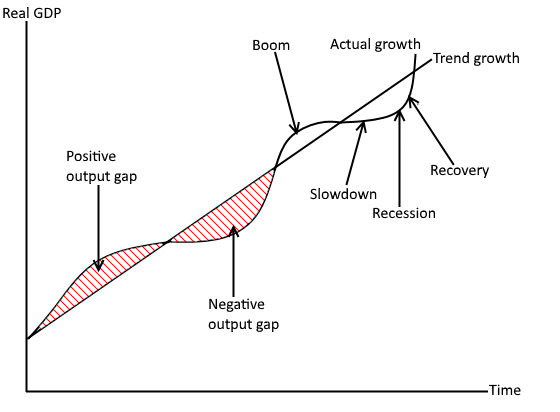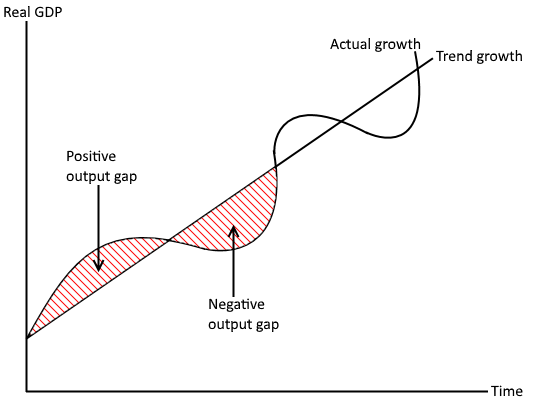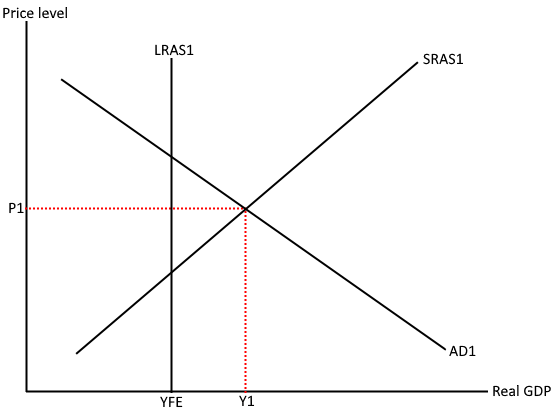# Output gaps

A) Distinction between actual growth rates and long-term trends in growth rates

Growth rate

This is measured by changes in real GDP over time. The growth rate is often very volatile as shown on any economic cycle diagram. This is demonstrated by the various booms and recessions that take place as shown from the diagram below. Although this is the case, some countries try to reduce these fluctuations through the use of automatic stabilisers. If the actual growth rate is above the trend growth there is a positive output gap and sometimes a boom. In this period there will be high levels of inflation due to lots of pressure on existing factors of production, caused by the economy operating past the level of full employment. On the other hand, when the actual growth rate is below the trend growth rate there is a negative output gap and sometimes a recession. In this period there will be low inflation due to little pressure on existing factors of production which is caused by the economy operating below full employment.

Long term trends in growth rates

This is the average sustainable rate of growth over a period of time, also known as the trend rate of growth. The trend of the long run growth rate is determined by changes in the productive capacity of the economy.B) Understanding of positive and negative output gaps and the difficulties of measurement

The output gap is the difference between the actual level of real GDP and the maximum potential level of real GDP. If the actual level of real GDP is less than the maximum potential level of real GDP there is a negative output gap, meaning there is spare capacity within the economy. On the other hand, if the actual level of real GDP is greater than the maximum potential level of real GDP then there is a positive output gap. Positive output gaps are unsustainable in the long run according to the classical economic model of AS/AD due to the fact that workers will revise up their wages in the long run causing SRAS to decrease and the economy to move back to full employment. During periods when there is a positive output within the economy, there are likely to be low levels of unemployment and high levels of inflation. The opposite characteristics are likely to take place during periods when there is a negative output gap (low inflation and high levels of unemployment).

It is very difficult to estimate the size of the output gap of an economy, due to the fact that in order to do this you need to estimate the economy’s maximum potential output level. The many different variables that determine the maximum potential output level make this difficult to do e.g. changes in the quantity of labour, the productivity/quality of the workforce, the spare capacity of individual businesses etc. All of this data has the potential to be inaccurate and therefore the figure for the maximum potential output of the economy is likely to be a very rough estimate as there lots of knowledge gaps and the data that economists do have to work with can be inaccurate.

C) Use of an AD/AS diagram to illustrate an output gap (level of spare capacity) in an economy

Economic cycle positive and negative output gapsWhen the actual growth rate is greater than the trend growth rate, there is a positive output gap within the economy. In contrast to this, when the actual growth rate is lower than the trend growth rate, there is a negative output gap within the economy.

Classical negative output gapYFE. This means the spare capacity within the economy is the difference between Y1 and YFE. As the output of YFE is greater than Y1 there is a negative output gap within the economy.

Classical positive output gapCurrent output is at Y1 and the price level within the economy is at P1. The full employment output of the economy is at YFE, which is less than the current output of the economy (Y1).